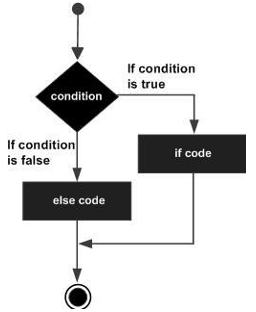# TypeScript - IfŌĆ”else Statement

An if can be followed by an optional else block. The else block will execute if the Boolean expression tested by the if statement evaluates to false.

## Syntax

```if(boolean_expression) {
// statement(s) will execute if the boolean expression is true
} else {
// statement(s) will execute if the boolean expression is false
}
```

## FlowchartThe if block guards the conditional expression. The block associated with the if statement is executed if the Boolean expression evaluates to true.

The if block may be followed by an optional else statement. The instruction block associated with the else block is executed if the expression evaluates to false.

## Example: Simple ifŌĆ”else

```var num:number = 12;
if (num % 2==0) {
console.log("Even");
} else {
console.log("Odd");
}
```

On compiling, it will generate the following JavaScript code −

```//Generated by typescript 1.8.10
var num = 12;
if (num % 2 == 0) {
console.log("Even");
} else {
console.log("Odd");
}
```

The above example prints whether the value in a variable is even or odd. The if block checks the divisibility of the value by 2 to determine the same. Here is the output of the above code −

```Even
```
typescript_decision_making.htm